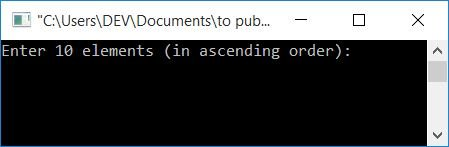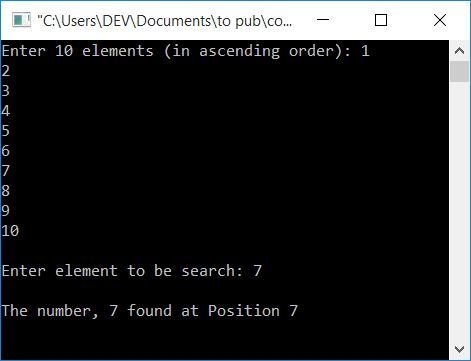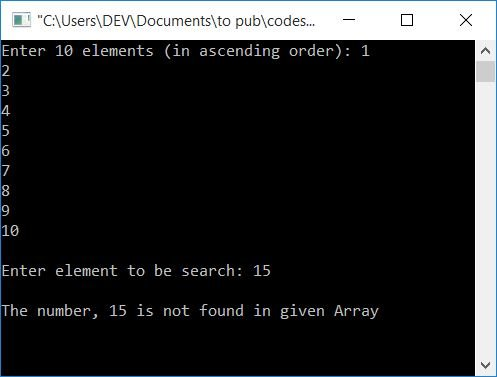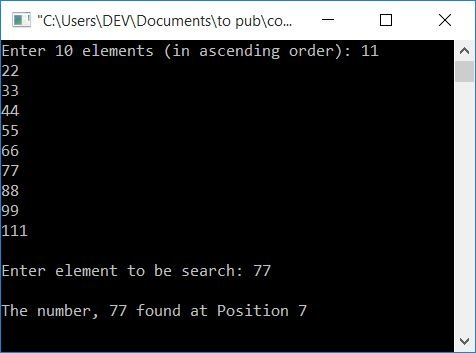# C Program for Binary Search

In this article, you'll learn and get code about how to search for an element in a given array using the binary search technique. But before going through the program, if you are not aware of how binary search works, then I recommend that you go through the step-by-step workings of binary search..

The following is a list of the programs you will go through, along with a step-by-step explanation:

## Binary Search in C

This is the simplest program for a binary search. In the most basic sense, we have asked the user to enter 10 elements or numbers without specifying the size of the array and then enter the required number of elements. Also, the sorting code block is not included in this program. So I've just asked to enter an already-sorted array as input. Let's take a look at the program:

```#include<stdio.h>
#include<conio.h>
int main()
{
int i, arr, search, first, last, middle;
printf("Enter 10 elements (in ascending order): ");
for(i=0; i<10; i++)
scanf("%d", &arr[i]);
printf("\nEnter element to be search: ");
scanf("%d", &search);
first = 0;
last = 9;
middle = (first+last)/2;
while(first <= last)
{
if(arr[middle]<search)
first = middle+1;
else if(arr[middle]==search)
{
printf("\nThe number, %d found at Position %d", search, middle+1);
break;
}
else
last = middle-1;
middle = (first+last)/2;
}
if(first>last)
getch();
return 0;
}```

This program was written in the Code::Blocks IDE. Here is the initial snapshot of the sample run:Now provide any 10 elements in ascending order, say 1, 2, 3, 4, 5, 6, 7, 8, 9, and 10, and then press the ENTER key. Again, enter any element or number to be searched, say 7, and press the ENTER key to see the output given in the snapshot here:If the user supplies all 10 numbers as entered in the above output, However, when he/she entered any number, say 15, to be searched from the given list of numbers, the following is the output:#### Program Explained

1. Declare all the required variables, say i, arr[], search, first, last, and middle of int (integer) type.
2. The size of arr[] is declared to be 10 in order to store up to ten elements, or numbers.
3. Now receive 10 numbers as input from the user.
4. As indexing in an array starts at 0, the first element gets stored in arr, the second element gets stored in arr, and so on.
5. Now, enter the number to be searched and save it in the search variable.
6. Now initialize 0 to the first (index), 9 to the last (index), and find the value of the middle (index) using first+last/2.
7. Create a while loop that continues running until the value of first (index) becomes less than or equal to the value of last (index).
8. The meaning of the above step is that the process inside the loop continues running until the interval becomes zero, as told in the logic given at the start of this article.
9. Inside the while loop, first check whether the value at the middle index (arr[middle]) is less than search (the number to be searched) or not using an if statement.
10. If it is, then initialize middle+1 to first and go to the last statement of the loop, that is, middle = (first+last)/2.
11. If the 9th step returns a false result, proceed to the next steps.
12. The program flow now moves to the else-if section, which checks whether the element in the middle (arr[middle]) is equal to the search (the number to be searched) or not.
13. If it is equal, then print the position. Here we have increased the index number by one to display the position of the number. As indexing starts at 0. For example, in an array, there are 4 numbers, say 10, 20, 30, and 40. As a result, the index will be 0, 1, 2, and 3. But normally people know it like this: 10, 20, 30, and 40 are present at first, second, third, and fourth positions.

14. So i have added 1 to the middle and printed its value as the position of the given number in the array.
15. Now the program flow goes to the last statement of the while loop, which is middle = (first+last)/2;.
16. If the 12th step evaluates to false, the else block is evaluated, and middle-1 is assigned to last, and the loop is repeated until the condition of the while loop evaluates to false.

Here is the modified version of the binary search program (as given above) in C. We've left the size of the array to the user to decide at runtime. And before performing the binary search, bubble sort is used to sort the given array in ascending order.

Important: This program finds the position of a number from a sorted array, not from the actual array that is entered by the user at run-time. For example, if the user supplies 10, 50, 20, 30, and 40 as array elements, and 20 as the number to search, Then this program sorts the array, so the array becomes 10, 20, 30, 40, and 50, and the position of 20 will be 2.

```#include<stdio.h>
#include<conio.h>
int main()
{
int i, j, n, arr, search, first, last, middle, temp;
printf("How many element you want to store in array ? ");
scanf("%d", &n);
printf("\nEnter %d array elements: ", n);
for(i=0; i<n; i++)
scanf("%d", &arr[i]);
printf("\nEnter element to be search: ");
scanf("%d", &search);

// sorting given array using bubble sort
for(i=0; i<(n-1); i++)
{
for(j=0; j<(n-i-1); j++)
{
if(arr[j]>arr[j+1])
{
temp = arr[j];
arr[j] = arr[j+1];
arr[j+1] = temp;
}
}
}

// sort array
printf("\nNow the sorted Array is:\n");
for(i=0; i<n; i++)
printf("%d ", arr[i]);

// back to binary search
first = 0;
last = n-1;
middle = (first+last)/2;
while(first <= last)
{
if(arr[middle]<search)
first = middle+1;
else if(arr[middle]==search)
{
printf("\n\nThe number, %d found at Position %d", search, middle+1);
break;
}
else
last = middle-1;
middle = (first+last)/2;
}
if(first>last)
getch();
return 0;
}```

Here is a snapshot of the sample run:### Binary search program in C using user-defined functions

Modify the first program in this article that does the same job, but this time using the function as shown in the program given below:

```#include<stdio.h>
#include<conio.h>
int binarySearchFun(int arr[], int);
int main()
{
int i, arr, search, pos;
printf("Enter 10 elements (in ascending order): ");
for(i=0; i<10; i++)
scanf("%d", &arr[i]);
printf("\nEnter element to be search: ");
scanf("%d", &search);
pos = binarySearchFun(arr, search);
if(pos==0)
else
printf("\nThe number, %d found at Position %d", search, pos);
getch();
return 0;
}
int binarySearchFun(int arr[], int search)
{
int first, last, middle;
first = 0;
last = 9;
middle = (first+last)/2;
while(first <= last)
{
if(arr[middle]<search)
first = middle+1;
else if(arr[middle]==search)
{
return (middle+1);
}
else
last = middle-1;
middle = (first+last)/2;
}
return 0;
}```

This is a snapshot of the sample output produced after running the above program:#### Program Explained

• In the above program, inside the function binarySearchFun(), if the number gets found or the condition arr[middle]==search evaluates to be true, then middle+1 gets returned to the function binarySearchFun() as its return value and this function gets terminated.
• The return value of this function gets initialized to the pos variable inside the main() function.
• Otherwise, if none of the elements (from the array) matched the given number (to be searched), then after exiting from the while loop from the binarySearchFun() function, the `return 0;` statement gets executed, and 0 gets returned and initialized to the pos variable inside the main() function.
• Now use the if-else case (in the main() function) to check and print whether the number is found or not.
• If found, then print its position, otherwise, print as "number is not found."

### Binary Search Program in C using Recursion

This is the last program on binary search. This program used a recursive function to find the number from the given array using the binary search technique. Let's take a look at the program:

```#include<stdio.h>
#include<conio.h>
int binarySearchRecFun(int [], int, int, int);
int main()
{
int i, arr, search, pos;
printf("Enter 10 elements (in ascending order): ");
for(i=0; i<10; i++)
scanf("%d", &arr[i]);
printf("\nEnter element to be search: ");
scanf("%d", &search);
pos = binarySearchRecFun(arr, 0, 9, search);
if(pos==0)
else
printf("\nThe number, %d found at Position %d", search, pos);
getch();
return 0;
}
int binarySearchRecFun(int arr[], int first, int last, int search)
{
int middle;
if(first>last)
return 0;
middle = (first+last)/2;
if(arr[middle]==search)
return (middle+1);
else if(arr[middle]>search)
binarySearchRecFun(arr, first, middle-1, search);
else if(arr[middle]<search)
binarySearchRecFun(arr, middle+1, last, search);
}```

You will get the same output as shown in the output of the above program, that is, a binary search using the function.

#### The same program in different languages

C Quiz

« Previous Program Next Program »